# Alternate dosing event syntax

A fun syntax for creating event objects for different dosing regimens.

events
Author

Kyle Baron

Published

January 1, 2019

``````library(mrgsolve)
library(dplyr)
options(mrgsolve.soloc="build")``````

I got into pharmacometrics after studying and working as a pharmacist, both in a community pharmacy (interning while I was a pharmacy student) and in an inpatient (hospital) pharmacy (practicing as a pharmacist while studying pharmacometrics and clinical pharmacology). So I always thought it would be cool to have mrgsolve take in dosing information like you would see on a Rx or written in a chart

``sig: 100 mg po daily x 7``

This post introduces something new in mrgsolve 0.9.0 that works along those lines.

# 1 Review

First, a review: we have these things called event objects in mrgsolve: they are simple ways to specify a part of a dosing regimen. They are meant to be quick and easy to create and you are able to combine different “elementary” objects together according to certain rules to make more complex dosing regimens.

For example, say we want a dose every day for one week. Assuming that our model is in hours, we could write

``````e1 <- ev(amt = 100, ii = 24, until = 24*7)

e1``````
``````Events:
time amt ii addl cmt evid
1    0 100 24    6   1    1``````
``mod <- modlib("pk1", delta=0.1) %>% Req(CP)``
``Building pk1 ... done.``
``mrgsim_e(mod, e1, end = 24*9) %>% plot``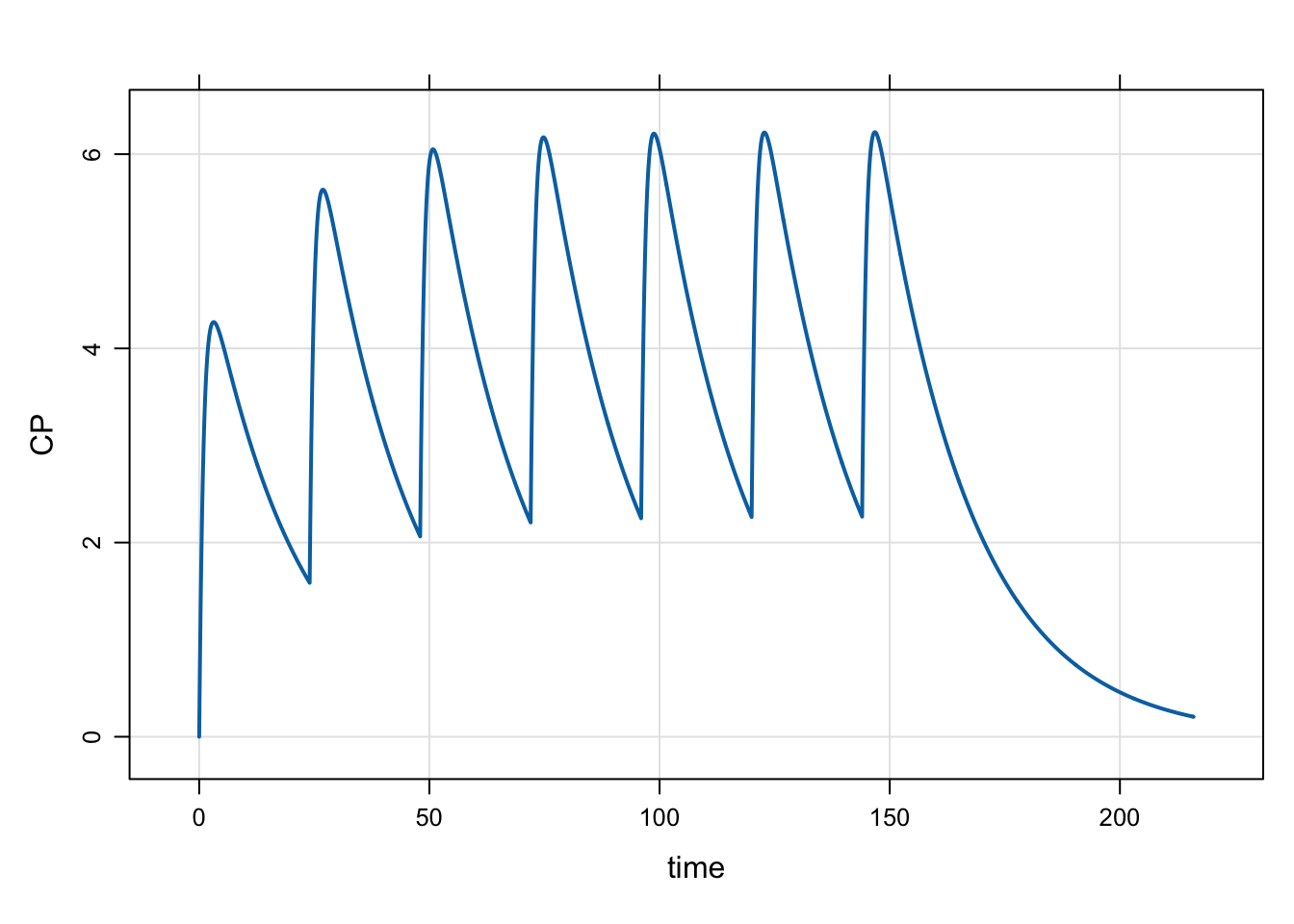And if I wanted to follow that by a week at every 48 hours, first create the q48h event object

``````e2 <- ev(amt = 100, ii = 48, until = 24*7)
e2``````
``````Events:
time amt ii addl cmt evid
1    0 100 48    3   1    1``````

and then combine them together in a sequence

``````e <- seq(e1,e2)

e``````
``````Events:
time amt ii addl cmt evid
1    0 100 24    6   1    1
2  168 100 48    3   1    1``````
``mrgsim_e(mod, e, end = 168*3) %>% plot``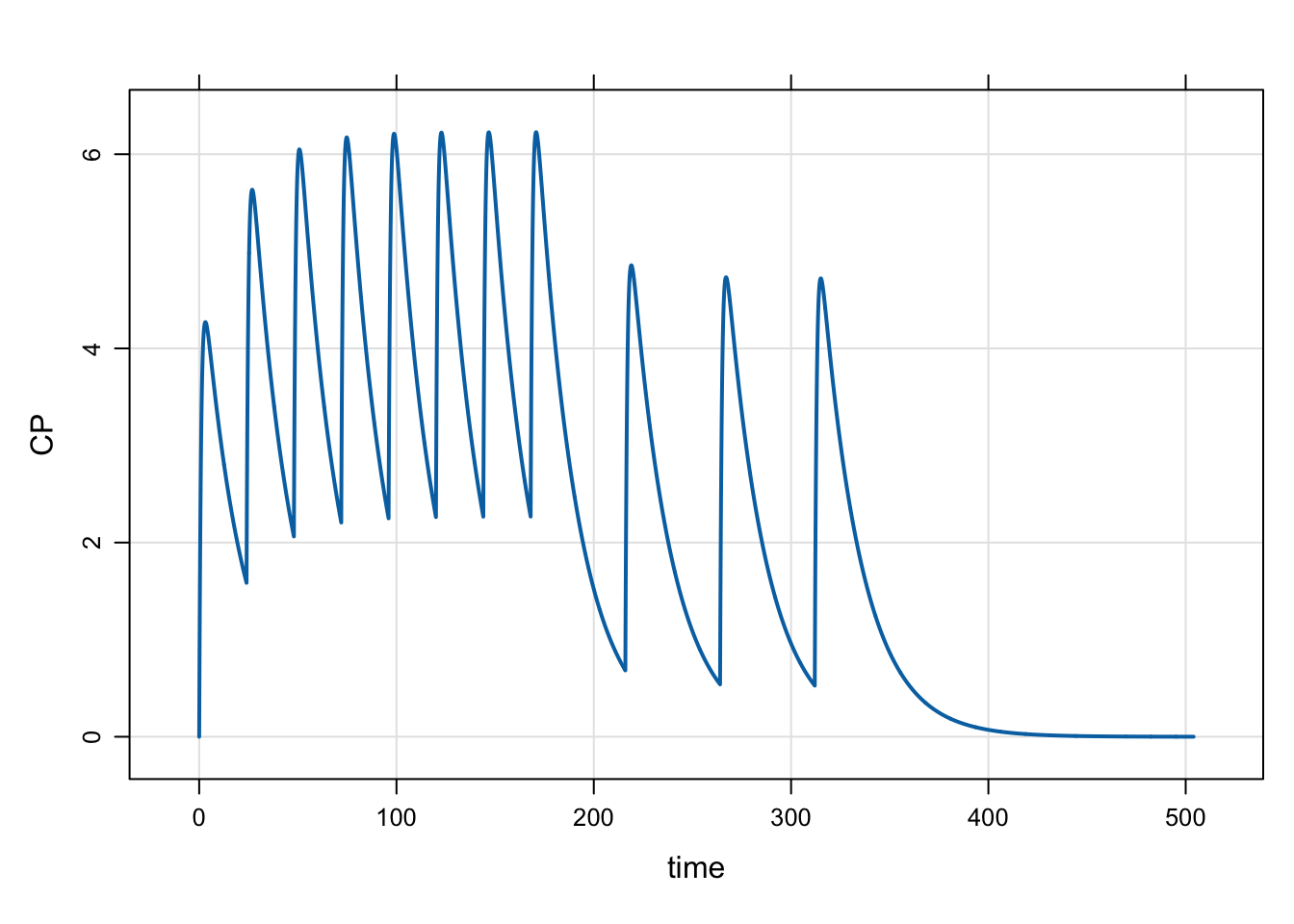And then if we wanted to repeat 3 cycles of that

``````e4 <- ev_repeat(e, n=3, as.ev = TRUE)

mrgsim_e(mod, e4, end = 168*8) %>% plot``````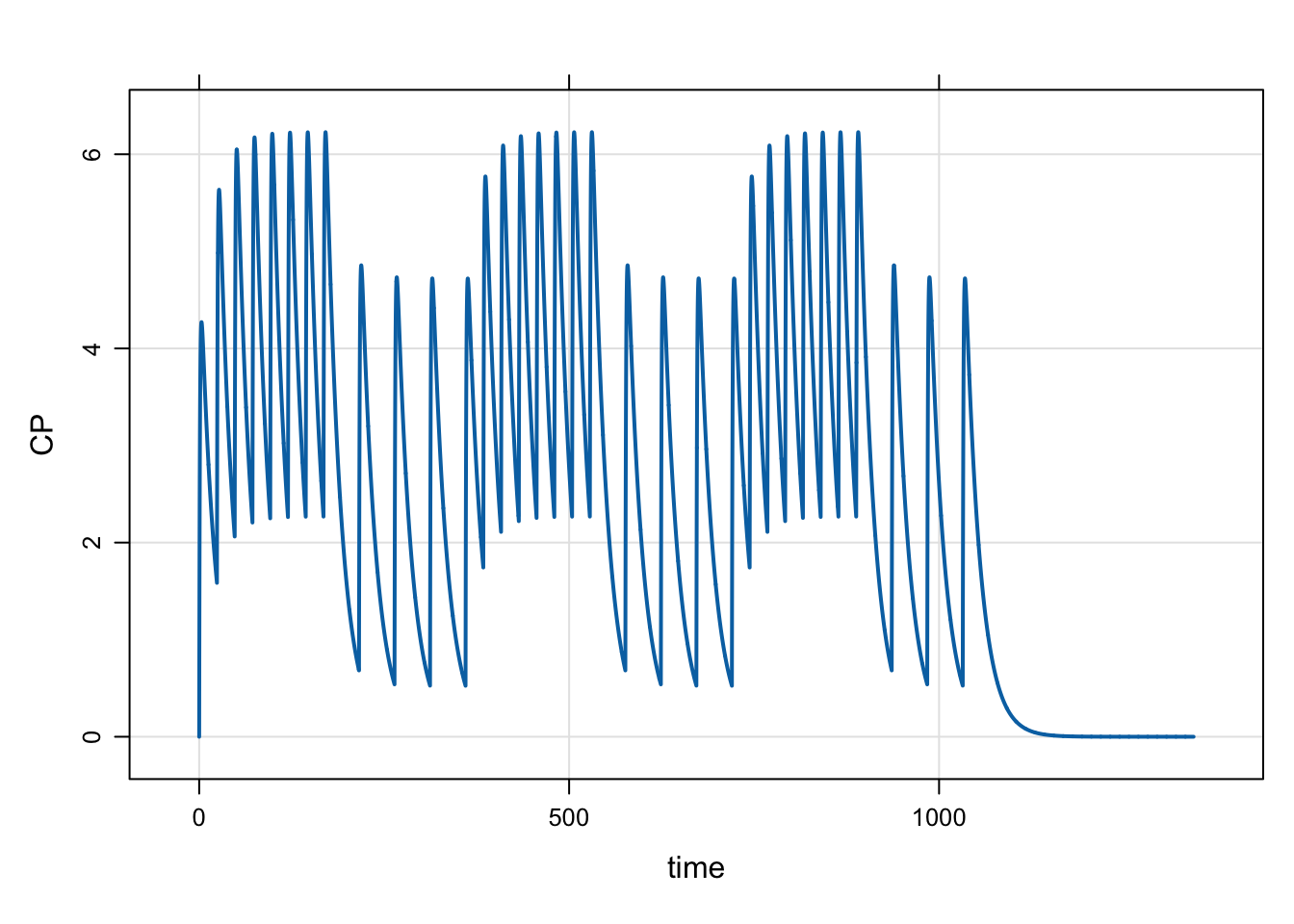# 2 New in 0.9.0

New in 0.9.0 is a grammar of specifying some of this dose event information. I’ve been wanting to do something like this for a while, but never quite had the right implementation. The current try isn’t perfect, but I thought it was worth it to give a try and see if we can improve.

## 2.1 Bolus doses

``````e5 <- ev_rx("100 q 24 x7 then 100 q48 x 4")

e5``````
``````Events:
time amt ii addl cmt evid
1    0 100 24    6   1    1
2  168 100 48    3   1    1``````
``mrgsim_e(mod, e5, end = 168*3) %>% plot()``The syntax is:

• Start with `amt`
• Dosing interval follows `q`
• Total number of doses follows `x`
• A second part of the sequence follows `then`

## 2.2 Infusion

To do an infusion, the duration of the infusion comes after `over`

``````e6 <- ev_rx("100 over 10 q 48 x 3")

e6``````
``````Events:
time amt rate ii addl cmt evid
1    0 100   10 48    2   1    1``````
``mrgsim_e(mod, e6, end = 168) %>% plot()``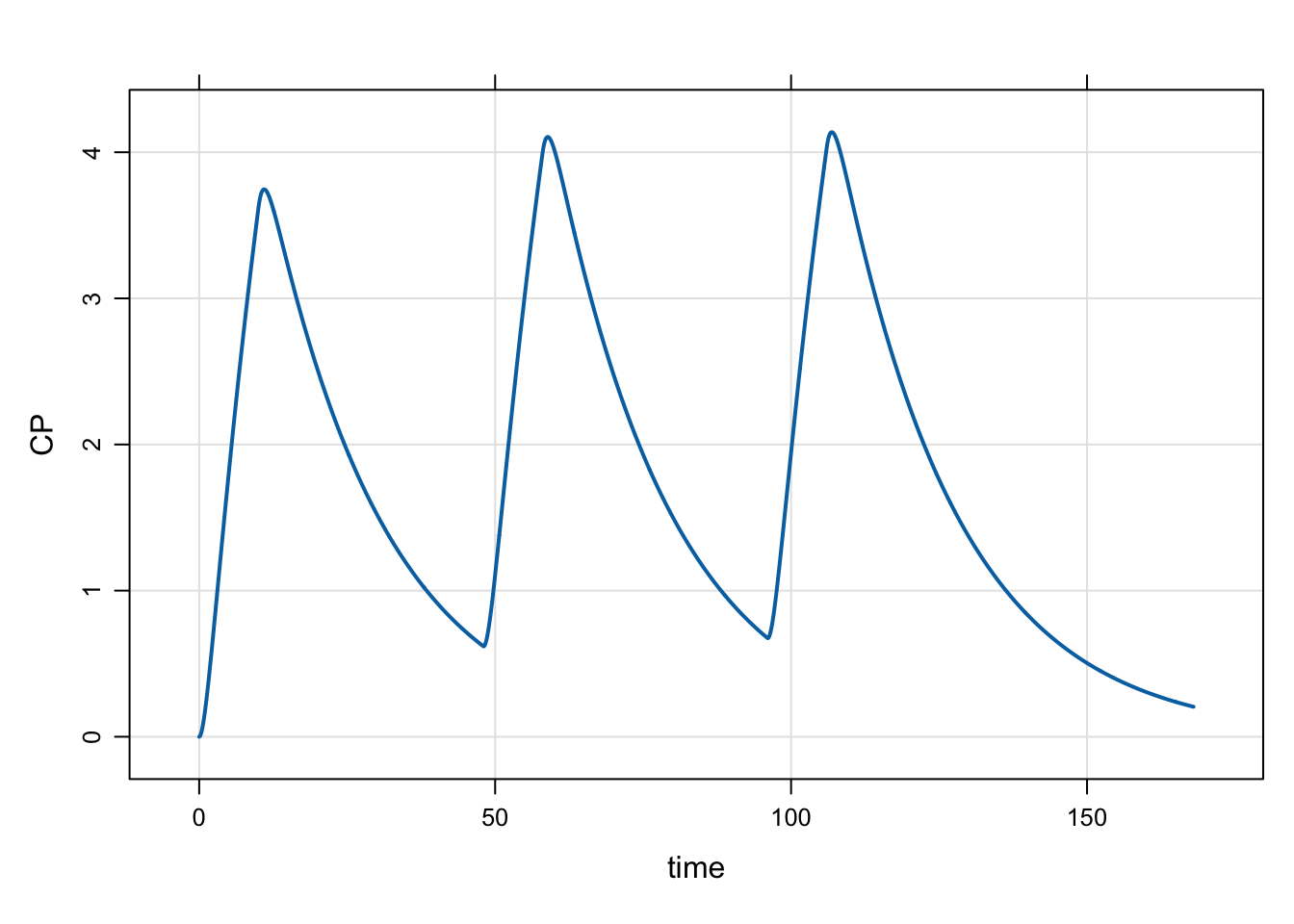Hrm … but we wanted the infusion to be IV, not in the depot compartment. To do that, put the compartment number after `in`

``````e7 <- ev_rx("100 over 10 in 2 q 48 x 3")

e7``````
``````Events:
time amt rate ii addl cmt evid
1    0 100   10 48    2   2    1``````
``mrgsim_e(mod,e7, end = 168) %>% plot()``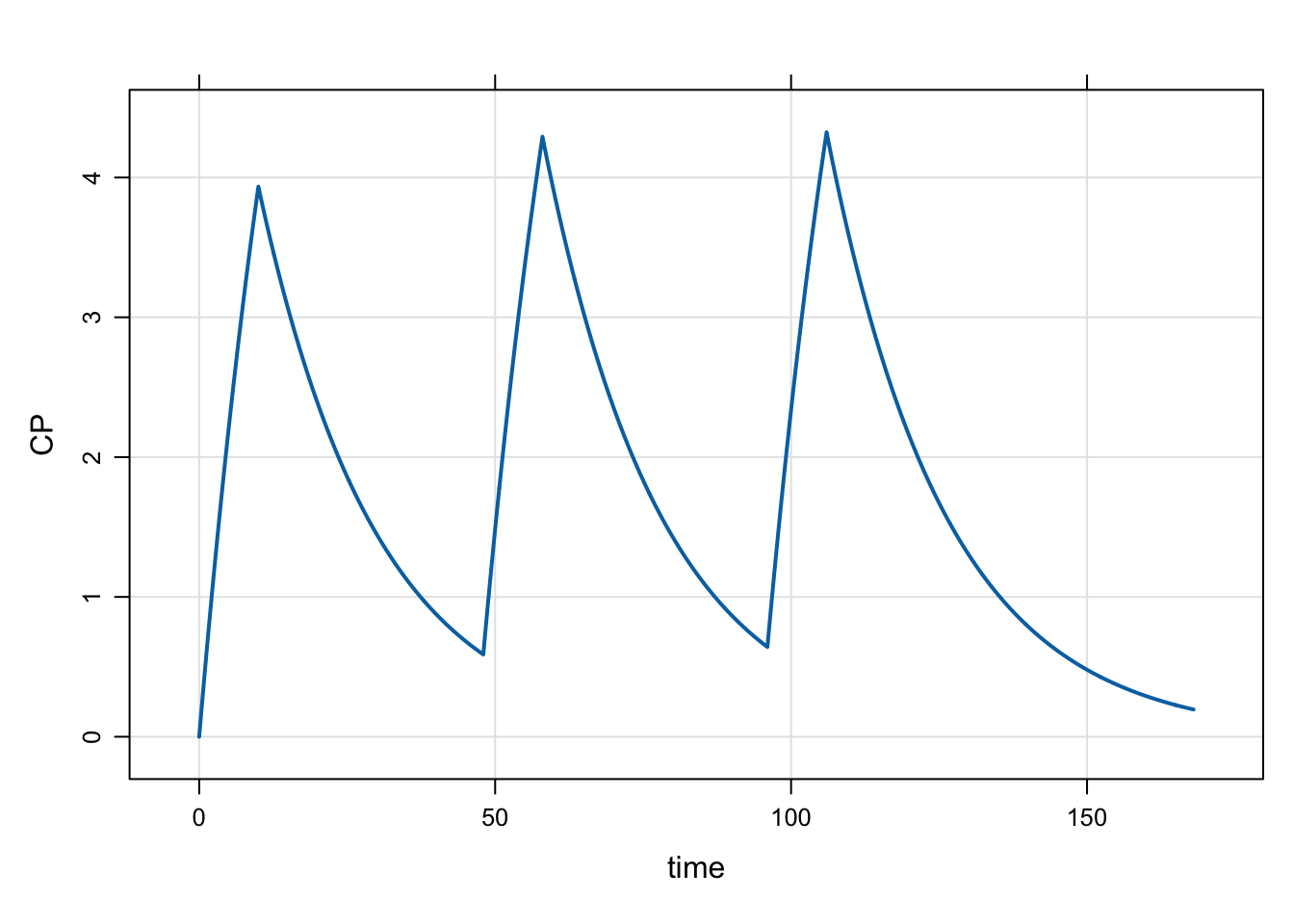## 2.3 Another sequence

Rather than `then` you can separate by `comma`. Also, see if you can tell what `after` does here:

``````e <- ev_rx("100 q 24 x 7, 50 q 12 x 14, 200 q48 x 3 after 72")

e``````
``````Events:
time amt ii addl cmt evid
1    0 100 24    6   1    1
2  168  50 12   13   1    1
3  408 200 48    2   1    1``````

It still takes a fair amount of text to get all of this out, but hopefully the notation is relatively compact and expressive.

``mrgsim_e(mod, e, end = 600) %>% plot()``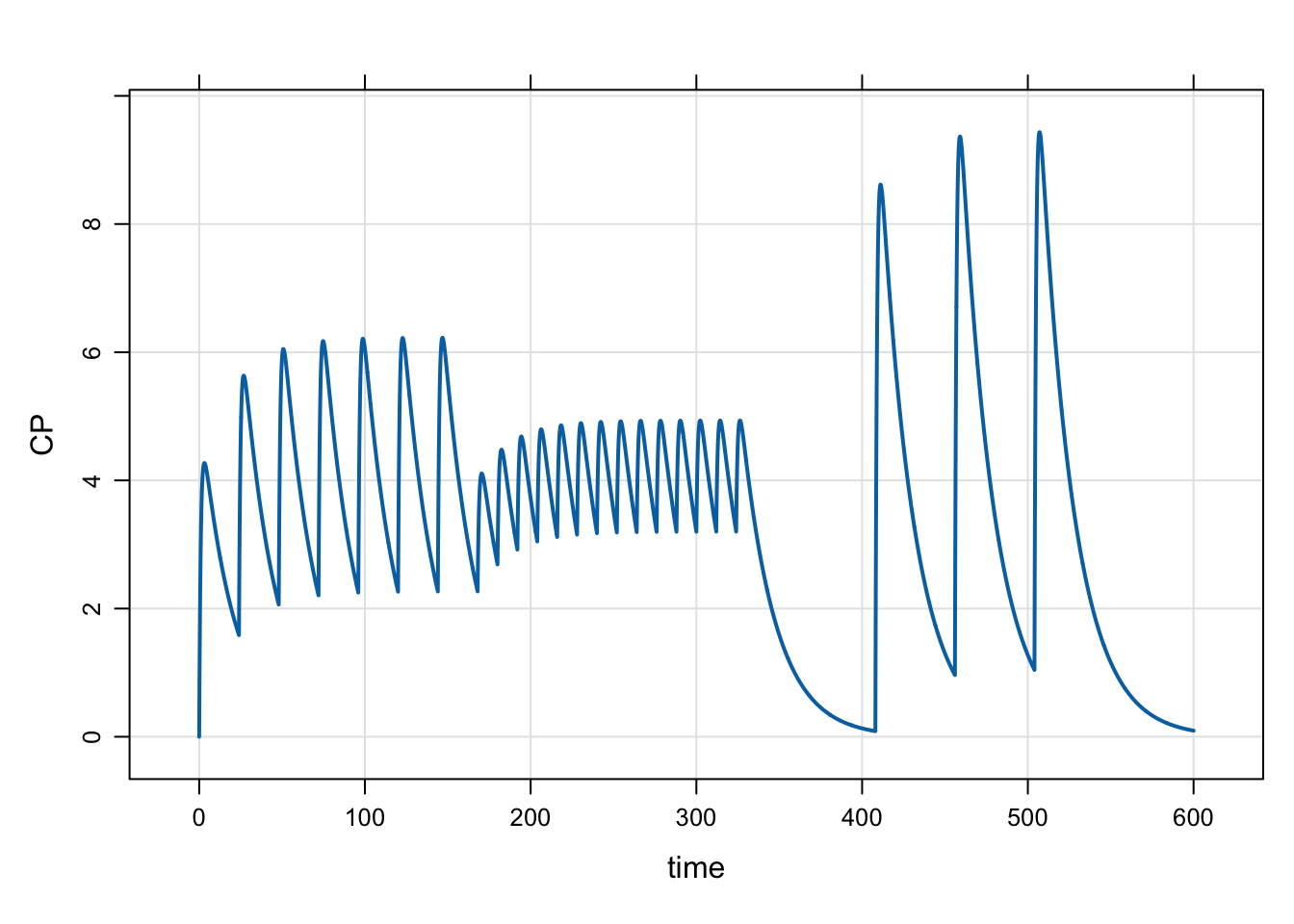# 3 Important note

I don’t see this ever replacing the regular event object construction. Maybe it is force of habit for me, but I still regularly just use the constructor. That might be easier in the end. For now, this alternate syntax is sort of proof of concept to see how it might work. Definitely you can’t do everything you might want to do with this syntax. In that case, just revert to the usual constructors.